# College Physics with Mastering Physics

Physics & Astronomy

## Quiz 4 : Force and MotionStudy FlashcardsLooking for Introductory Physics Homework Help?

## Quiz 4 :Force and Motion

Question Type(a) If an object is at rest, there must be no forces acting on it. Is this statement correct Explain. (b) If the net force on an object is zero, can you conclude that the object is at rest Explain.
Free
Essay

(a)
No. The statement is not true.
There are many forces acting on an object at rest. The weight of the object is always downwards and many other forces like normal force, frictional force, push or pull may act on it. According to Newton's second law of motion, the object remains at rest when the net force acting on it is zero.
(b)
No. If the net force is zero, then according to Newton's second law of motion, the acceleration of the object is zero. Thus, the object may move with a constant velocity or be at rest. Therefore, the net force acting on the object is zero doesn't conclude that it is at rest. It can be in motion with a constant velocity.

Tags
Choose question tagAn object weighs 300 N on Earth and 50 N on the Moon. Does the object also have less inertia on the Moon
Free
Essay

The weight of an object on Earth is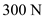and its weight on Moon is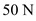.
Mass of a body is a numerical measure of inertia. This gives that a massive body offers more inertia, or more resistance to a change in motion than a less massive body. Hence, the inertia does not depend on the weight of the body. So, the object on the Earth and on the Moon has same inertia.

Tags
Choose question tagA hockey puck with a weight of 0.50 lb is sliding freely across a section of very smooth (frictionless) horizontal ice. (a) When it is sliding freely, how does the upward force of the ice on the puck (the normal force) compare with the upward force when the puck is sitting permanently at rest: (I) The upward force is greater when the puck is sliding; (2) the upward force is less when it is sliding; (3) the upward force is the same in both situations (b) Calculate the upward force on the puck in both situations.
Free
Essay

From the Newton's second law of motion, the net force acting on the system is equal to rate of change in momentum.
In other word, the net force acting on the system is equal to the product of the mass of the system and the acceleration of the system.
The expression for the net force acting on the system is as follows: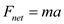Here, m is the mass of the system.
(a)
Case-1: The hockey puck is sliding freely in the horizontal direction.
In the case-1, there is no net force acting on the puck in vertical direction.
Hence, the normal force is equal to the weight of the puck.
Case-2: The hockey puck is at rest.
In the case-2, there is no net force acting on the puck in vertical direction.
Hence, the normal force is equal to the weight of the puck.
Therefore, the upward force acting on the hockey puck is same in the both cases.
Thus, option (3) is correct.
(b)
Calculate the upward force acting on the hockey puck in the both cases as follows:
In both cases, the upward force on the puck is same i.e., normal force.
Hence, the normal force acting on the puck in the both cases is nothing but weight of the puck.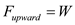The weight of the puck is as follows: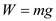Substitute 0.50 lb for m and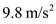for g in the equationand solve for W.Therefore, the upward force acting on the hockey puck in the both cases is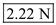.

Tags
Choose question tagAs a follow-up to Conceptual Question, consider a child holding a helium balloon in a closed car at rest. What would the child observe when the car (a) accelerates from rest and (b) brakes to a stop (The balloon does not touch the roof of the car.) Conceptional Question Consider an air-bubble level that is sitting on a horizontal surface (Fig.). Initially, the air bubble is in the middle of the horizontal glass tube. (a) If the level is pushed and a force is applied to accelerate it, which way would the bubble move Which way would the bubble move if the force is then removed and the level slows down, due to friction (b) Such a level is sometimes used as an "accelerometer" to indicate the direction of the acceleration. Explain the principle involved. [ Hint : Think about pushing a pan of water.]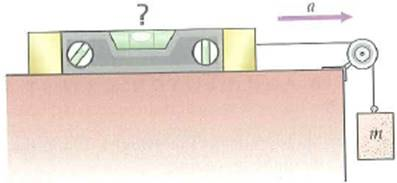FIGURE An air-bubble level/accelerometer See Conceptual Question.
Essay
Tags
Choose question tagConsider a 2.0-kg ball and a 6.0-kg ball in free fall. (a) What is the net force acting on each (b) What is the acceleration of each
Essay
Tags
Choose question tagConsider an air-bubble level that is sitting on a horizontal surface (Fig.). Initially, the air bubble is in the middle of the horizontal glass tube. (a) If the level is pushed and a force is applied to accelerate it, which way would the bubble move Which way would the bubble move if the force is then removed and the level slows down, due to friction (b) Such a level is sometimes used as an "accelerometer" to indicate the direction of the acceleration. Explain the principle involved. [ Hint : Think about pushing a pan of water.]FIGURE An air-bubble level/accelerometer See Conceptual Question.
Essay
Tags
Choose question tagMass is related to an object's (a) weight, (b) inertia, (c) density, (d) all of the preceding.
Tags
Choose question tagThe following is an old trick (Fig.). If a tablecloth is yanked out very quickly, the dishes on it will barely move. WhyFIGURE Magic or physics See Conceptual Question.
Essay
Tags
Choose question tagIf an object is moving at constant velocity, (a) there must be a force in the direction of the velocity, (b) there must be no force in the direction of the velocity, (c) there must- be no net force, (d) there must be a net force in the direction of the velocity.
Tags
Choose question tagA net force of 4.0 N gives an object an acceleration of 10 m/s 2. What is the mass of the object
Essay
Tags
Choose question tagIf the net force on an object is zero, the object could (a) be at rest, (b) be in motion at a constant velocity, (c) have zero acceleration, (d) all of the preceding.
Tags
Choose question tagWhen on a jet airliner that is taking off, you feel that you are being "pushed" back into the seat. Use Newton's first law to explain why.
Essay
Tags
Choose question tagWhich has more inertia, 20 cm 3 of water or 10 cm 3 of aluminum, and how many times more (See Table 9.2.)
Essay
Tags
Choose question tagThe force required to keep a rocket ship moving at a constant velocity in deep space is (a) equal to the weight of the ship, (b) dependent on how fast the ship is moving, (c) equal to that generated by the rocket's engines at half power, (d) zero.
Tags
Choose question tagA 5.0-kg block at rest on a frictionless surface is acted on by forces F 1 = 5.5 N and F 2 = 3.5 N as illustrated in Fig. What additional force will keep the block at rest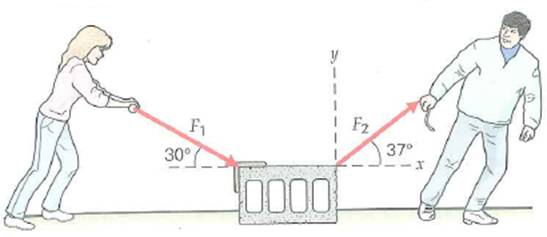FIGURE Two applied forces See Exercise.
Essay
Tags
Choose question tagTwo forces act on a 5.0-kg object sitting on a friction-less horizontal surface. One force is 30 N in the + x -direction, and the other is 35 N in the x -direction. What is the acceleration of the object
Essay
Tags
Choose question tagA force (a) always produces motion, (b) is a scalar quantity, (c) is capable of producing a change in motion, (d) both a and b.
Tags
Choose question tagIn Exercise, if the 35-N force acted downward at an angle of 40° relative to the horizontal, what would be the acceleration in this case Exercise Two forces act on a 5.0-kg object sitting on a friction-less horizontal surface. One force is 30 N in the + x -direction, and the other is 35 N in the x -direction. What is the acceleration of the object
Essay
Tags
Choose question tagAnother old one: Referring to Fig., (a) how would you pull to get the upper string to break (b) Flow would you pull to get the lower string to break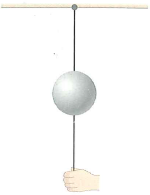FIGURE Give it a pull. See Conceptual Question.
Essay